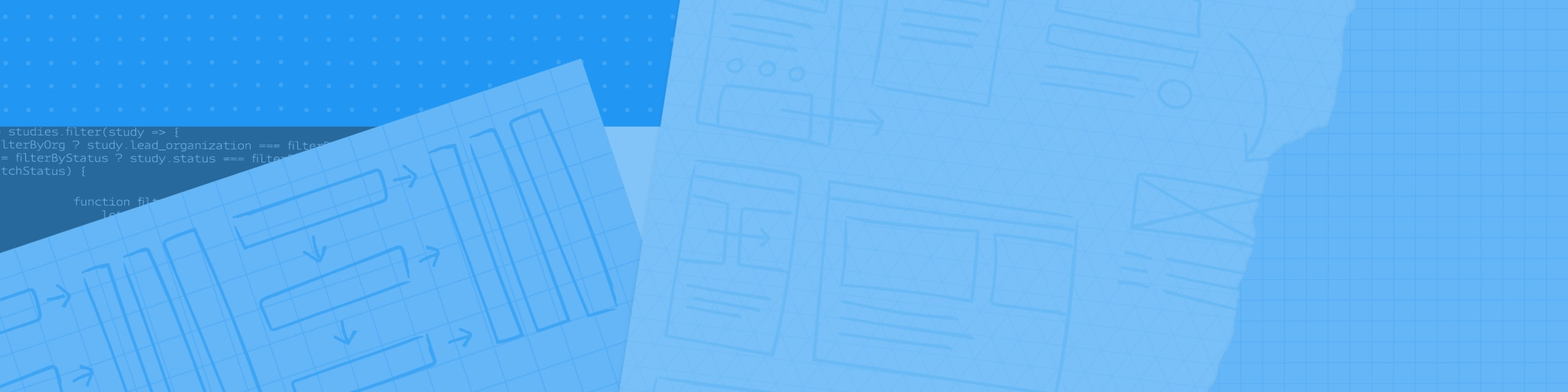# Machine Learning Workshop ft. Kaggle Competition/CodeLabs

Join GDG Cloud Silicon Valley for a hands-on workshop where you will solve machine learning problems from beginning-to-end using Logistic Regression. Logistic regression is a statistical method for analyzing a dataset in which there are one or more independent variables that determine an outcome. The outcome is measured with a dichotomous variable (in which there are only two possible outcomes).

RSVP'd

### Key Themes

Join GDG Cloud Silicon Valley for a hands-on workshop where you will solve machine learning problems from beginning-to-end using Logistic Regression.

Logistic regression is a statistical method for analyzing a dataset in which there are one or more independent variables that determine an outcome.

The outcome is measured with a dichotomous variable (in which there are only two possible outcomes). We would use Logistic Regression algorithms to determine if passengers on Titanic survived or died based on their attributes.

In this workshop you can expect to:

1) Get a quick overview of Machine Learning.
2) Get an environment set on your laptop to learn machine learning.
3) Walkthrough "Titanic" example from beginning to end.

• What to bring
Laptop with wifi capability and Chrome installed

# Organizers

• ## LeopoldoHernandez

Wayfair

GDG Organizer

• ## DanielGoncharov

GDG Organizer